Courses

# Compressibility & Consolidation - 1

## 10 Questions MCQ Test Mock Test Series for Civil Engineering (CE) GATE 2020 | Compressibility & Consolidation - 1

Description
This mock test of Compressibility & Consolidation - 1 for Civil Engineering (CE) helps you for every Civil Engineering (CE) entrance exam. This contains 10 Multiple Choice Questions for Civil Engineering (CE) Compressibility & Consolidation - 1 (mcq) to study with solutions a complete question bank. The solved questions answers in this Compressibility & Consolidation - 1 quiz give you a good mix of easy questions and tough questions. Civil Engineering (CE) students definitely take this Compressibility & Consolidation - 1 exercise for a better result in the exam. You can find other Compressibility & Consolidation - 1 extra questions, long questions & short questions for Civil Engineering (CE) on EduRev as well by searching above.
QUESTION: 1

### Consider the following statements: 1. Coefficient of consolidation normally increases with decreasing liquid limit of clay. 2. The larger the value of coefficient of consolidation, the longer it takes for full consolidation to occur. Which of these statements is/are correct?

Solution:

From Taylor’s formula, we have,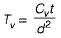For full consolidation i.e. U= 100%. will more or less be a constant.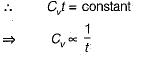Thus, larger the value of coefficient of consolidation, smaller it takes for full consolidation to occur.

QUESTION: 2

### The virgin compression curve for a soil is shown in figure given below. What is the compression index of the soil?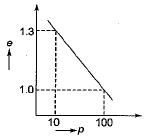Solution:

Compression index is given by the equation,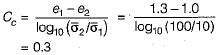QUESTION: 3

### The e-p curve for a soil is shown in the figure below. The coefficient of compressibility (in m2kN) of the soil is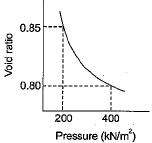Solution:

Coefficient of compressibility,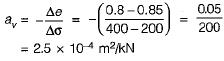QUESTION: 4

The figure given shows the state of a sample of clay before and after consolidation. Based on these figures, the settlement of a clay layer of initial thickness H will be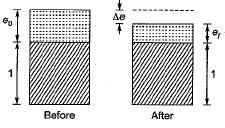Solution: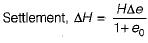QUESTION: 5

The settlement analysis for a clay layer draining from top and bottom shows a settlement of 2.5 cm in 4 years and an ultimate settlement of 10 cm. However detailed subsurface investigation reveals that there is no drainage at the bottom. The ultimate settlement in this condition will be

Solution:

Ultimate settlement does not depend upon drainage condition.

QUESTION: 6

Match List-1 with List-11 and select the correct answer using the codes given below the lists (notations have their usual meaning):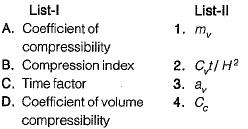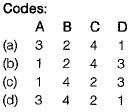Solution:
QUESTION: 7

The liquid limit of a saturated normally consolidated soil is 59%. The compression index of this soil for the virgin compression curve will be

Solution: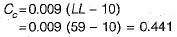QUESTION: 8

Coefficient of consolidation is used to calculate

Solution:

The average degree of consolidation depends upon the non-dimensional time factor Tv. The time factor Tv depends upon the coefficient of consolidation Cv, time t and drainage path d.

QUESTION: 9

The value of a compression index for remoulded sample, whose liquid limit is 50%, is

Solution:

For remoulded sample,
Cc = 0.007 ( LL - 10)
= 0.007 (50 - 10) = 0.28

QUESTION: 10

Rate of consolidation

Solution:

With increase in temperature viscosity of water decreases and coefficient of permeability increases. As coefficient of consolidation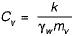∴ CV  increases and rate of consolidation increase.